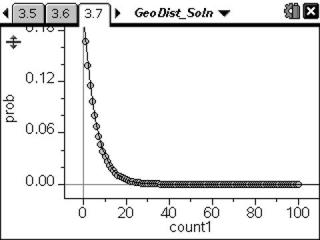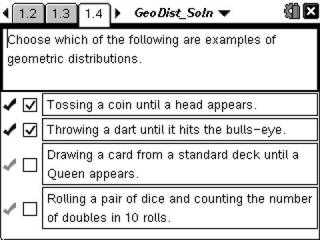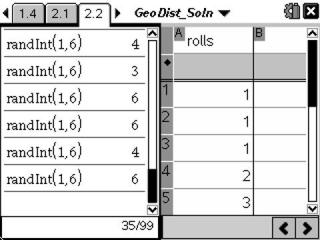# Activities

•##### Download

•• ##### Subject Area

• Math: Statistics: Normal Distributions
• Math: Statistics: Probability and Random Variables

• ##### Author9-12

45 Minutes

• ##### Device
• TI-Nspire™ Navigator™
• TI-Nspire™
• TI-Nspire™ CAS
• ##### Software

TI-Nspire™
TI-Nspire™ CAS

2.0

• ##### Report an Issue

Geometric Distributions#### Activity Overview

Students simulate a geometric distribution of rolling a die to determine experimental probabilities and calculate theoretical probabilities.

#### Key Steps

•Problem 1 introduces students to the geometric distribution. There are several self-check questions to test students understanding.

•In this problem, students will investigate the probability of the first 6 appearing on the fourth roll. Students will use the Random Integer command to simulate the geometric distribution. They will combine their results with 3 classmates and calculate the experimental probability.

•In Problem 3, students will explore the theoretical probabilities of a geometric distribution using the Geometric Pdf command. They will then derive the formula for calculating the probability of the first 6 appearing on the nth roll. Then students will graph the probabilities as a scatter plot and display the regression equation. They will algebraically verify that the formula and the regression equation are the same.